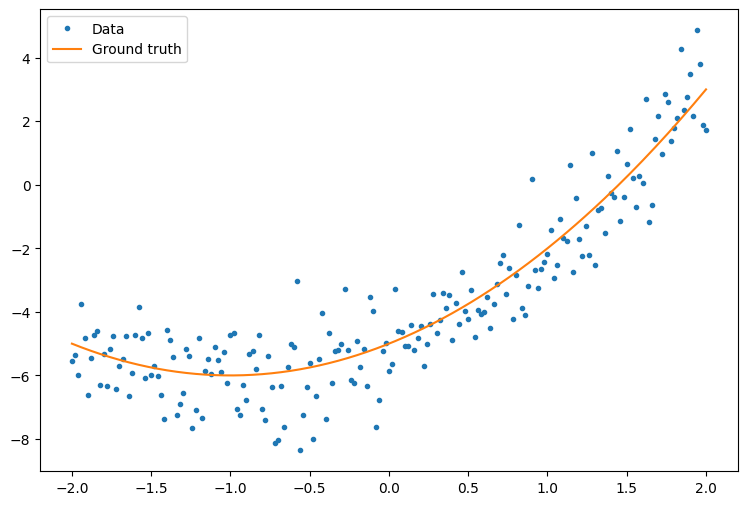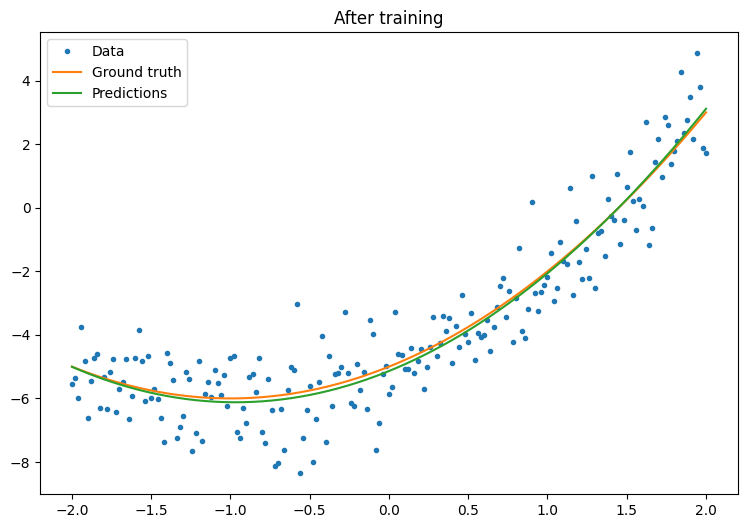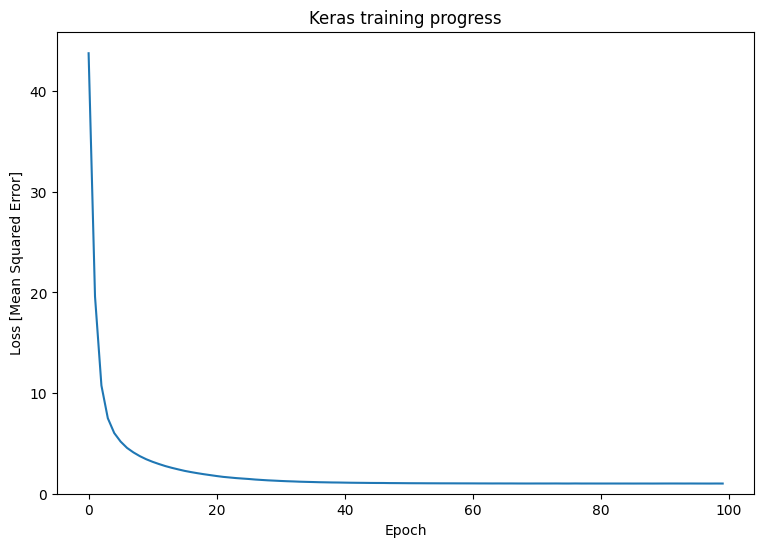Google I/O is a wrap! Catch up on TensorFlow sessions

# TensorFlow basics

This guide provides a quick overview of TensorFlow basics. Each section of this doc is an overview of a larger topic—you can find links to full guides at the end of each section.

TensorFlow is an end-to-end platform for machine learning. It supports the following:

• Multidimensional-array based numeric computation (similar to NumPy.)
• GPU and distributed processing
• Automatic differentiation
• Model construction, training, and export
• And more

## Tensors

TensorFlow operates on multidimensional arrays or tensors represented as `tf.Tensor` objects. Here is a two-dimensional tensor:

``````import tensorflow as tf

x = tf.constant([[1., 2., 3.],
[4., 5., 6.]])

print(x)
print(x.shape)
print(x.dtype)
``````
```tf.Tensor(
[[1. 2. 3.]
[4. 5. 6.]], shape=(2, 3), dtype=float32)
(2, 3)
<dtype: 'float32'>
```

The most important attributes of a `tf.Tensor` are its `shape` and `dtype`:

TensorFlow implements standard mathematical operations on tensors, as well as many operations specialized for machine learning.

For example:

``````x + x
``````
```<tf.Tensor: shape=(2, 3), dtype=float32, numpy=
array([[ 2.,  4.,  6.],
[ 8., 10., 12.]], dtype=float32)>
```
``````5 * x
``````
```<tf.Tensor: shape=(2, 3), dtype=float32, numpy=
array([[ 5., 10., 15.],
[20., 25., 30.]], dtype=float32)>
```
``````x @ tf.transpose(x)
``````
```<tf.Tensor: shape=(2, 2), dtype=float32, numpy=
array([[14., 32.],
[32., 77.]], dtype=float32)>
```
``````tf.concat([x, x, x], axis=0)
``````
```<tf.Tensor: shape=(6, 3), dtype=float32, numpy=
array([[1., 2., 3.],
[4., 5., 6.],
[1., 2., 3.],
[4., 5., 6.],
[1., 2., 3.],
[4., 5., 6.]], dtype=float32)>
```
``````tf.nn.softmax(x, axis=-1)
``````
```<tf.Tensor: shape=(2, 3), dtype=float32, numpy=
array([[0.09003057, 0.24472848, 0.6652409 ],
[0.09003057, 0.24472848, 0.6652409 ]], dtype=float32)>
```
``````tf.reduce_sum(x)
``````
```<tf.Tensor: shape=(), dtype=float32, numpy=21.0>
```

Running large calculations on CPU can be slow. When properly configured, TensorFlow can use accelerator hardware like GPUs to execute operations very quickly.

``````if tf.config.list_physical_devices('GPU'):
print("TensorFlow **IS** using the GPU")
else:
print("TensorFlow **IS NOT** using the GPU")
``````
```TensorFlow **IS** using the GPU
```

Refer to the Tensor guide for details.

## Variables

Normal `tf.Tensor` objects are immutable. To store model weights (or other mutable state) in TensorFlow use a `tf.Variable`.

``````var = tf.Variable([0.0, 0.0, 0.0])
``````
``````var.assign([1, 2, 3])
``````
```<tf.Variable 'UnreadVariable' shape=(3,) dtype=float32, numpy=array([1., 2., 3.], dtype=float32)>
```
``````var.assign_add([1, 1, 1])
``````
```<tf.Variable 'UnreadVariable' shape=(3,) dtype=float32, numpy=array([2., 3., 4.], dtype=float32)>
```

Refer to the Variables guide for details.

## Automatic differentiation

Gradient descent and related algorithms are a cornerstone of modern machine learning.

To enable this, TensorFlow implements automatic differentiation (autodiff), which uses calculus to compute gradients. Typically you'll use this to calculate the gradient of a model's error or loss with respect to its weights.

``````x = tf.Variable(1.0)

def f(x):
y = x**2 + 2*x - 5
return y
``````
``````f(x)
``````
```<tf.Tensor: shape=(), dtype=float32, numpy=-2.0>
```

At `x = 1.0`, `y = f(x) = (1**2 + 2*1 - 5) = -2`.

The derivative of `y` is `y' = f'(x) = (2*x + 2) = 4`. TensorFlow can calculate this automatically:

``````with tf.GradientTape() as tape:
y = f(x)

g_x = tape.gradient(y, x)  # g(x) = dy/dx

g_x
``````
```<tf.Tensor: shape=(), dtype=float32, numpy=4.0>
```

This simplified example only takes the derivative with respect to a single scalar (`x`), but TensorFlow can compute the gradient with respect to any number of non-scalar tensors simultaneously.

Refer to the Autodiff guide for details.

## Graphs and tf.function

While you can use TensorFlow interactively like any Python library, TensorFlow also provides tools for:

• Performance optimization: to speed up training and inference.
• Export: so you can save your model when it's done training.

These require that you use `tf.function` to separate your pure-TensorFlow code from Python.

``````@tf.function
def my_func(x):
print('Tracing.\n')
return tf.reduce_sum(x)
``````

The first time you run the `tf.function`, although it executes in Python, it captures a complete, optimized graph representing the TensorFlow computations done within the function.

``````x = tf.constant([1, 2, 3])
my_func(x)
``````
```Tracing.
<tf.Tensor: shape=(), dtype=int32, numpy=6>
```

On subsequent calls TensorFlow only executes the optimized graph, skipping any non-TensorFlow steps. Below, note that `my_func` doesn't print tracing since `print` is a Python function, not a TensorFlow function.

``````x = tf.constant([10, 9, 8])
my_func(x)
``````
```<tf.Tensor: shape=(), dtype=int32, numpy=27>
```

A graph may not be reusable for inputs with a different signature (`shape` and `dtype`), so a new graph is generated instead:

``````x = tf.constant([10.0, 9.1, 8.2], dtype=tf.float32)
my_func(x)
``````
```Tracing.
<tf.Tensor: shape=(), dtype=float32, numpy=27.3>
```

These captured graphs provide two benefits:

• In many cases they provide a significant speedup in execution (though not this trivial example).
• You can export these graphs, using `tf.saved_model`, to run on other systems like a server or a mobile device, no Python installation required.

Refer to Intro to graphs for more details.

## Modules, layers, and models

`tf.Module` is a class for managing your `tf.Variable` objects, and the `tf.function` objects that operate on them. The `tf.Module` class is necessary to support two significant features:

1. You can save and restore the values of your variables using `tf.train.Checkpoint`. This is useful during training as it is quick to save and restore a model's state.
2. You can import and export the `tf.Variable` values and the `tf.function` graphs using `tf.saved_model`. This allows you to run your model independently of the Python program that created it.

Here is a complete example exporting a simple `tf.Module` object:

``````class MyModule(tf.Module):
def __init__(self, value):
self.weight = tf.Variable(value)

@tf.function
def multiply(self, x):
return x * self.weight
``````
``````mod = MyModule(3)
mod.multiply(tf.constant([1, 2, 3]))
``````
```<tf.Tensor: shape=(3,), dtype=int32, numpy=array([3, 6, 9], dtype=int32)>
```

Save the `Module`:

``````save_path = './saved'
tf.saved_model.save(mod, save_path)
``````
```INFO:tensorflow:Assets written to: ./saved/assets
2022-01-19 02:29:48.135588: W tensorflow/python/util/util.cc:368] Sets are not currently considered sequences, but this may change in the future, so consider avoiding using them.
```

The resulting SavedModel is independent of the code that created it. You can load a SavedModel from Python, other language bindings, or TensorFlow Serving. You can also convert it to run with TensorFlow Lite or TensorFlow JS.

``````reloaded = tf.saved_model.load(save_path)
``````
```<tf.Tensor: shape=(3,), dtype=int32, numpy=array([3, 6, 9], dtype=int32)>
```

The `tf.keras.layers.Layer` and `tf.keras.Model` classes build on `tf.Module` providing additional functionality and convenience methods for building, training, and saving models. Some of these are demonstrated in the next section.

Refer to Intro to modules for details.

## Training loops

Now put this all together to build a basic model and train it from scratch.

First, create some example data. This generates a cloud of points that loosely follows a quadratic curve:

``````import matplotlib
from matplotlib import pyplot as plt

matplotlib.rcParams['figure.figsize'] = [9, 6]
``````
``````x = tf.linspace(-2, 2, 201)
x = tf.cast(x, tf.float32)

def f(x):
y = x**2 + 2*x - 5
return y

y = f(x) + tf.random.normal(shape=)

plt.plot(x.numpy(), y.numpy(), '.', label='Data')
plt.plot(x, f(x),  label='Ground truth')
plt.legend();
``````Create a model:

``````class Model(tf.keras.Model):
def __init__(self, units):
super().__init__()
self.dense1 = tf.keras.layers.Dense(units=units,
activation=tf.nn.relu,
kernel_initializer=tf.random.normal,
bias_initializer=tf.random.normal)
self.dense2 = tf.keras.layers.Dense(1)

def call(self, x, training=True):
# For Keras layers/models, implement `call` instead of `__call__`.
x = x[:, tf.newaxis]
x = self.dense1(x)
x = self.dense2(x)
return tf.squeeze(x, axis=1)
``````
``````model = Model(64)
``````
``````plt.plot(x.numpy(), y.numpy(), '.', label='data')
plt.plot(x, f(x),  label='Ground truth')
plt.plot(x, model(x), label='Untrained predictions')
plt.title('Before training')
plt.legend();
``````Write a basic training loop:

``````variables = model.variables

optimizer = tf.optimizers.SGD(learning_rate=0.01)

for step in range(1000):
prediction = model(x)
error = (y-prediction)**2
mean_error = tf.reduce_mean(error)

if step % 100 == 0:
print(f'Mean squared error: {mean_error.numpy():0.3f}')
``````
```Mean squared error: 16.123
Mean squared error: 0.997
Mean squared error: 0.964
Mean squared error: 0.946
Mean squared error: 0.932
Mean squared error: 0.921
Mean squared error: 0.913
Mean squared error: 0.907
Mean squared error: 0.901
Mean squared error: 0.897
```
``````plt.plot(x.numpy(),y.numpy(), '.', label="data")
plt.plot(x, f(x),  label='Ground truth')
plt.plot(x, model(x), label='Trained predictions')
plt.title('After training')
plt.legend();
``````That's working, but remember that implementations of common training utilities are available in the `tf.keras` module. So consider using those before writing your own. To start with, the `Model.compile` and `Model.fit` methods implement a training loop for you:

``````new_model = Model(64)
``````
``````new_model.compile(
loss=tf.keras.losses.MSE,
optimizer=tf.optimizers.SGD(learning_rate=0.01))

history = new_model.fit(x, y,
epochs=100,
batch_size=32,
verbose=0)

model.save('./my_model')
``````
```INFO:tensorflow:Assets written to: ./my_model/assets
```
``````plt.plot(history.history['loss'])
plt.xlabel('Epoch')
plt.ylim([0, max(plt.ylim())])
plt.ylabel('Loss [Mean Squared Error]')
plt.title('Keras training progress');
``````Refer to Basic training loops and the Keras guide for more details.

[]
[]